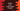# Python program to print a right angled triangle## Python 3 program to print a right angled triangle :

In this tutorial, we will learn how to print one right-angled triangle using python 3. A triangle is called a right-angled triangle if it’s one angle is of 90 degrees or right angle.

The other two angles depend on the side length of the triangle.

If the sides making the right angle are equal or if the triangle is an isosceles triangle, the other two angles will always 45 degrees. If the sides are not equal, then the other two angles will be different.

In this tutorial, we will create one isosceles right-angled triangle, i.e. one angle is right angle, and the other two angles are 45 degrees.

Printing a right-angled triangle is an easier task than printing any other type of triangles programmatically. In this blog post, I will show you step by step on solving this problem programmatically.

The output will look as like below :

``````1
2 2
3 3 3
4 4 4 4
5 5 5 5 5
6 6 6 6 6 6``````

As you can see, we are actually printing the rows and columns here. By using loops, we can achieve results like this. We will run two loops. One for printing rows and one for columns.

For the first row, we need to print one column, two columns for the second row, three for the third row, etc.

You can use any loop (for or while) you want. In this example, we will use ‘for’ loop.

The outer loop will run for 6 times in this example. Let’s say it runs for i from 1 to 6. The inner-loop will be used to print the numbers: it will run for 1 to i.

• For i = 1 for outer loop, j will run for 1 for inner loop
• For i = 2 for outer loop, j will run for 1,2 for inner loop
• For i = 3 for outer loop, j will run for 1,2,3 for inner loop
• For i = 4 for outer loop, j will run for 1,2,3,4 for inner loop
• For i = 5 for outer loop, j will run for 1,2,3,4,5 for inner loop
• For i = 6 for outer loop, j will run for 1,2,3,4,5,6 for inner loop

For each time the inner loop runs, we will print the value of ‘i’. So, for i = 1, it will print only 1 , for i = 2 , it will print only 2 etc.

Also, the inner loop will run for ‘i’ amount of time. For the first row, it will run for one time, two times for the second row, three for the third row etc. On each iteration of the inner loop, we are printing the character.

### Python Program :

``````#example 1
height = int(input("Enter the height of the triangle :"))
for i in range(1,height+1):
for j in range(1,i+1):
print(str(i)+" ", end='')
print()``````

You can copy the programs from here.

### Output:

The above program can be changed to print any type of right-angled triangle. e.g. you can print one right-angled triangle with the first row as ‘1’, second row as ‘1,2’, third row as ‘1,2,3’ etc.

Or you can use any other character to print the triangle like we are explaining below :

### Python program to print a right angle triangle using star (*) or any character :

We can modify the above program to print this triangle with any character we want :

``````#example 2
height = int(input("Enter the height of the triangle : "))
c = str(input("Enter the character you want to print the triangle : "))
for i in range(1,height+1):
for j in range(1,i+1):
print(c+" ", end='')
print()``````

### Sample Outputs :

As you can see, we can print triangles with any character that we want. Try to run the above program and drop one comment below if you have any queries.